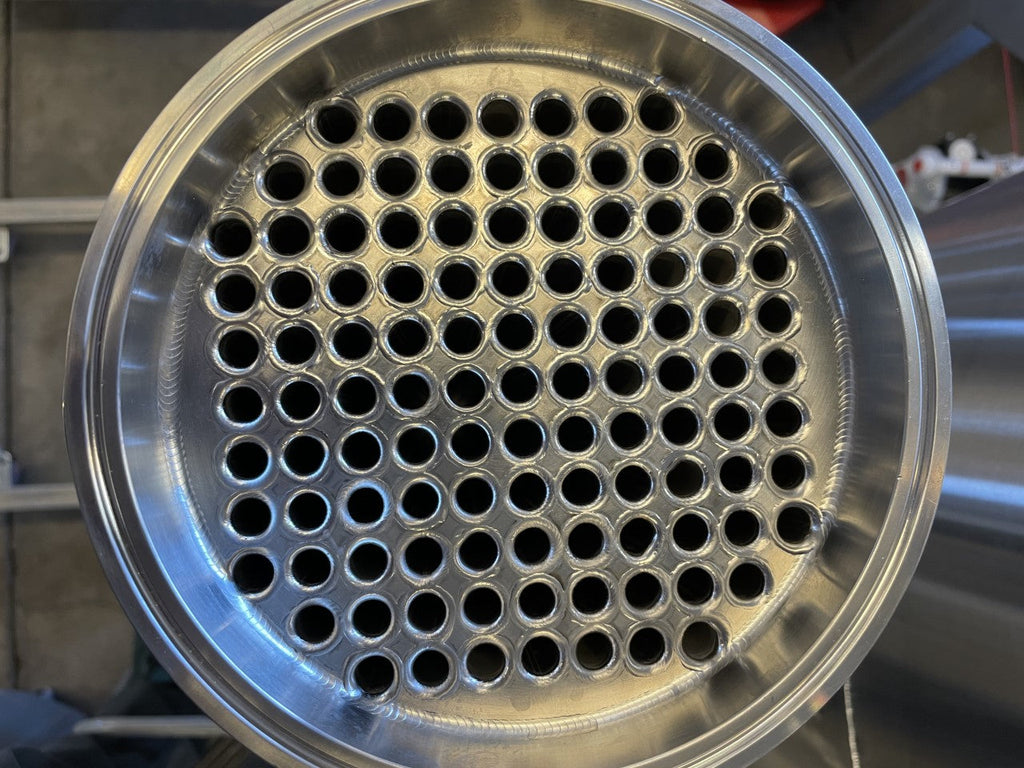# Stainless Steel Tube in Shell Condensers: Design, Fabrication, and Efficiency CalculationTube in shell condensers are a popular choice for heat transfer applications in a variety of industries, including chemical processing, pharmaceuticals, and food and beverage. These units consist of a bundle of tubes housed inside a shell, with a fluid flowing through the tubes and another fluid or gas flowing around the tubes in the shell.

Heat transfer in tube in shell condensers occurs through a combination of convection and conduction. The design of the unit is critical for achieving optimal heat transfer efficiency, and there are several factors to consider when designing and fabricating these units.

Design Criteria

When designing a tube in shell condenser, it is essential to consider the following criteria:

1. Heat transfer requirements: The heat transfer requirements will dictate the size of the unit, the number of tubes required, and the type of fluid used in the tubes and the shell.

2. Material compatibility: The materials used for the tubes and the shell should be compatible with the fluids and gases flowing through the unit.

3. Pressure requirements: The pressure requirements will determine the thickness of the tubes and the shell, as well as the type of sealing mechanism used.

4. Flow rate: The flow rate of the fluids and gases is a critical parameter that affects the heat transfer efficiency of the unit.

5. Temperature fluctuations: Temperature fluctuations in the fluids and gases can affect the efficiency of the unit, so it is essential to design the unit to accommodate these fluctuations.

Fabrication Challenges

The fabrication of tube in shell condensers can be challenging due to the complexity of the design and the materials used. The most significant challenges include:

1. Tube-to-tube sheet welding: Welding the tubes to the tube sheet requires precise welding techniques to prevent leaks.

2. Material handling: The materials used in tube in shell condensers are heavy and difficult to handle, requiring specialized equipment and safety measures.

3. Quality control: Quality control is critical during fabrication to ensure that the unit meets the design criteria and will function correctly.

Efficiency Calculation

The efficiency of a tube in shell condenser is calculated using the following parameters:

1. Heat transfer coefficient: The heat transfer coefficient is a measure of the rate of heat transfer between the fluids and gases.

2. Overall heat transfer coefficient: The overall heat transfer coefficient takes into account the heat transfer coefficient of both the shell and the tube sides.

3. Fouling factor: Fouling is the accumulation of deposits on the tube and shell surfaces, which reduces heat transfer efficiency. The fouling factor is a measure of how much fouling occurs over time.

4. Temperature difference: The temperature difference between the inlet and outlet fluids and gases affects the heat transfer efficiency.

5. Flow rate: The flow rate of the fluids and gases affects the heat transfer efficiency.

Calculating the inlet/outlet temperatures and flow rates for the shell side of a tube in shell condenser is crucial to ensuring efficient heat transfer. The following equations can help you determine the required parameters:

Q = m * Cp * ΔT

where: Q = Heat transfer rate (kW) m = Mass flow rate (kg/s) Cp = Specific heat capacity (kJ/kg.K) ΔT = Temperature difference (K)

To calculate the required inlet/outlet temperatures and flow rates, you can rearrange this equation as follows:

m = Q / (Cp * ΔT)

This equation allows you to calculate the mass flow rate of the shell side fluid required to achieve the desired heat transfer rate.

In addition to calculating the mass flow rate, you will also need to determine the inlet/outlet temperatures of the shell side fluid. To do this, you can use the following equation:

Tout = Tin - (Q / (m * Cp))

where: Tout = Outlet temperature (°C) Tin = Inlet temperature (°C)

This equation allows you to calculate the outlet temperature of the shell side fluid based on the heat transfer rate, mass flow rate, and specific heat capacity of the fluid.

It is important to note that these equations are only applicable for steady-state conditions. In dynamic conditions, additional factors such as the thermal inertia of the system and time-dependent changes in the inlet temperature and flow rate may need to be considered.

By using these equations, you can calculate the required inlet/outlet temperatures and flow rates for the shell side of a tube in shell condenser, which is critical for achieving efficient heat transfer in your application.

Weight of Finished Units

The weight of a tube in shell condenser can vary depending on the size and materials used. Typically, these units are relatively heavy due to the materials used in their construction. To minimize weight, manufacturers may use thinner materials, but this must be balanced against the need for sufficient thickness to handle the required pressure.

Conclusion

Tube in shell condensers are an essential component of many heat transfer applications, and designing and fabricating these units requires careful consideration of several critical factors. By understanding the design criteria, fabrication challenges, efficiency calculation, and inlet/outlet temperature and flow rate calculations, you can select the right tube in shell condenser for your application.